# Visually understanding multiplying fractions and whole numbers

##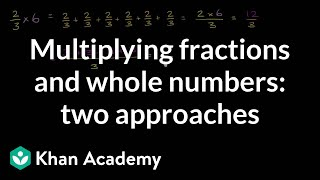By Khan Academy

When faced with multiplying whole numbers and fractions, understanding these two approaches will help solve the problem.# Visually understanding multiplying fractions and whole numbers

##By Khan Academy

When faced with multiplying whole numbers and fractions, understanding these two approaches will help solve the problem.# Visually understanding multiplying fractions and whole numbers

##By Khan Academy

When faced with multiplying whole numbers and fractions, understanding these two approaches will help solve the problem.# [4.NF.4b-1.0] Multiplying Fractions - Common Core Standard - Word Problem

##By Freckle education

Understand a multiple of a/b as a multiple of 1/b, and use this understanding to multiply a fraction by a whole number# Multiplying Fractions by Whole Numbers

##By Skubes ed

Understand a multiple of a/b as a multiple of 1/b, and use this understanding to multiply a fraction by a whole number# Unit Fractions and Multiples of Fractions

##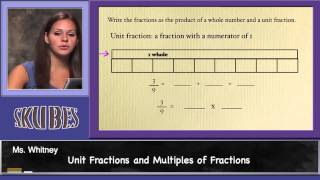By Skubes ed

Understand a multiple of a/b as a multiple of 1/b, and use this understanding to multiply a fraction by a whole number.# Multiplying Fractions by Whole Numbers

##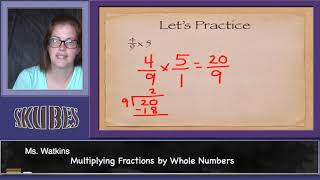By Skubes ed

Understand a multiple of a/b as a multiple of 1/b, and use this understanding to multiply a fraction by a whole number.# [4.NF.4b-1.0] Multiplying Fractions - Common Core Standard

##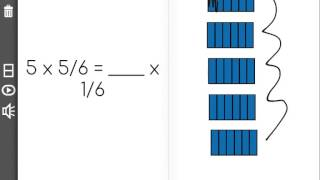By Freckle education

Understand a multiple of a/b as a multiple of 1/b, and use this understanding to multiply a fraction by a whole number# [3.NBT.1-1.0] Rounding to 10/100 - Common Core Standard

##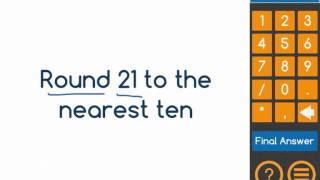By Front Row

Use place value understanding to round whole numbers to the nearest 10# Adding Fractions with Like Denominators

##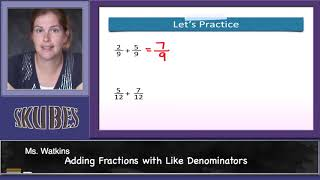By Skubes ed

Understand addition and subtraction of fractions as joining and separating parts referring to the same whole# [4.NF.3a-1.0] Intro to Fraction Addition - Common Core Standard - Word Problem

##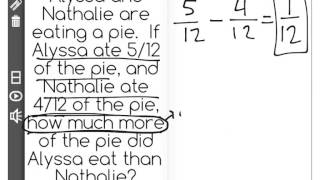By Freckle education

Understand addition and subtraction of fractions as joining and separating parts referring to the same whole# [4.NF.3a-1.0] Intro to Fraction Addition - Common Core Standard

##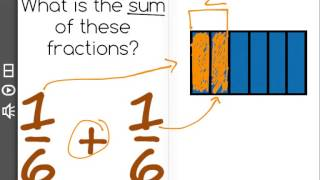By Freckle education

Understand addition and subtraction of fractions as joining and separating parts referring to the same whole# [4.NF.3a-1.0] Intro to Fraction Addition - Common Core Standard - Word Problem

##By Freckle education

Understand a fraction a/b with a greater than 1 as a sum of fractions 1/b. Understand addition and subtraction of fractions as joining and separating parts referring to the same whole.# [4.NF.3a-1.0] Intro to Fraction Addition - Common Core Standard

##By Freckle education

Understand a fraction a/b with a greater than 1 as a sum of fractions 1/b. Understand addition and subtraction of fractions as joining and separating parts referring to the same whole.# Visually understanding multiplying fractions and whole numbers

##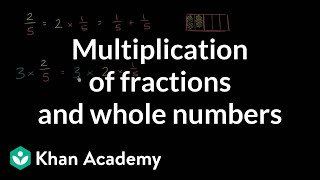By Khan Academy

Here we have a nice visual explanation of how a mixed number is really a multiplication of a fraction and a whole number.# Visually understanding multiplying fractions and whole numbers

##By Khan Academy

Here we have a nice visual explanation of how a mixed number is really a multiplication of a fraction and a whole number.# Visually understanding multiplying fractions and whole numbers

##By Khan Academy

Here we have a nice visual explanation of how a mixed number is really a multiplication of a fraction and a whole number.# Understanding multiplying fractions by fractions

##By Khan Academy

When faced with multiplying whole numbers and fractions, understanding these two approaches will help solve the problem.# Rounding: Nearest 10 or 100 | Good to Know | WSKG

##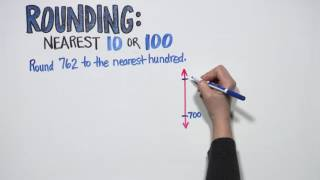By WSKG Public Media

(3.NBT.1) Use place value understanding to round whole numbers to the nearest 10 or 100.# Understanding multiplying fractions by fractions

##By Khan Academy

When faced with multiplying whole numbers and fractions, understanding these two approaches will help solve the problem.